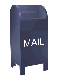## Lattice Pricing in Java

Lattice pricing is a technique for arriving at prices for options (e.g., call and put options) and for other financial instruments, like fixed income securities. Lattice pricing is covered in a number of text books including Options and Futures and other Derivatives by John Hull and Investment Science by David Luenberger. The Oxford guide to financial modeling By Thomas S. Y. Ho and Sang-bin Yi (which can be found, in part, in Google Books) has some very clear discussions on lattice pricing. The discussion on using a lattice to price a chooser option is very good.

The code published here does both lattice pricing and lattice pricing of what's called a "chooser option". This code to price the chooser option is based on the lattice pricing code. The chooser option code was written to answer an exercise in Luenberger's Investment Science (1998): Chapter 12, exercise 15.

A year after I wrote this code I took a course in options and derivatives where I wrote a code that priced an exotic Call option called a Shout option.

This algorithm follows exactly the lattices that you see in text books. In writing the code (in R, this time, not Java) I realized that the algorithm used here is very inefficient in both memory use and computation.

What I later learned from pricing the Shout option is that an option price that is exact to four decimal places takes about 1000 time steps (for an option with a exercise period of one year). The computational limitations become apparent with this many time steps.

So if I were to write this algorithm today (and I was not working under a homework deadline) I would use a much more efficient algorithm.

You're welcome to do what you want with this code, as long as you agree that I have no liability for your using this code (e.g., if you bring down the banking system, its on your head, not mine).

• BinomialLattice.java This is the basic binomial lattice code. It calculates strike prices, calls, European puts and American Puts.

• ChooserLattice.java This is the binomial lattice code with the addition of code to calculate the pricing of a "chooser option" which allows you to convert the option to either a put or a call at some predefined point in the future.

• lattice.tar The above source code as a tar archive

• lattice.jar The above source code as a Java jar file

Ian Kaplan
November, 2011
Last updated: January, 2013